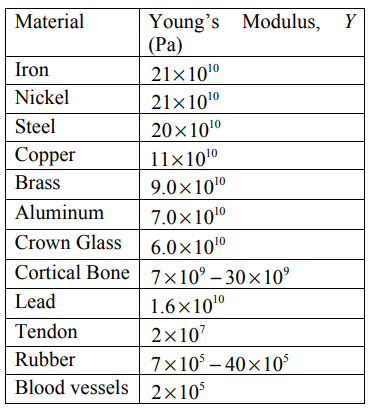# 26.2: Stress and Strain in Tension and Compression

$$\newcommand{\vecs}{\overset { \rightharpoonup} {\mathbf{#1}} }$$ $$\newcommand{\vecd}{\overset{-\!-\!\rightharpoonup}{\vphantom{a}\smash {#1}}}$$$$\newcommand{\id}{\mathrm{id}}$$ $$\newcommand{\Span}{\mathrm{span}}$$ $$\newcommand{\kernel}{\mathrm{null}\,}$$ $$\newcommand{\range}{\mathrm{range}\,}$$ $$\newcommand{\RealPart}{\mathrm{Re}}$$ $$\newcommand{\ImaginaryPart}{\mathrm{Im}}$$ $$\newcommand{\Argument}{\mathrm{Arg}}$$ $$\newcommand{\norm}{\| #1 \|}$$ $$\newcommand{\inner}{\langle #1, #2 \rangle}$$ $$\newcommand{\Span}{\mathrm{span}}$$ $$\newcommand{\id}{\mathrm{id}}$$ $$\newcommand{\Span}{\mathrm{span}}$$ $$\newcommand{\kernel}{\mathrm{null}\,}$$ $$\newcommand{\range}{\mathrm{range}\,}$$ $$\newcommand{\RealPart}{\mathrm{Re}}$$ $$\newcommand{\ImaginaryPart}{\mathrm{Im}}$$ $$\newcommand{\Argument}{\mathrm{Arg}}$$ $$\newcommand{\norm}{\| #1 \|}$$ $$\newcommand{\inner}{\langle #1, #2 \rangle}$$ $$\newcommand{\Span}{\mathrm{span}}$$$$\newcommand{\AA}{\unicode[.8,0]{x212B}}$$

Consider a rod with cross sectional area A and length $$l_{0}$$ Two forces of the same magnitude $$F_{\perp}$$ are applied perpendicularly at the two ends of the section stretching the rod to a length $$l$$ (Figure $$\PageIndex{1}$$), where the beam has been stretched by a positive amount $$\delta l=l-l_{0}$$.Figure $$\PageIndex{1}$$: Tensile stress on a rod

The ratio of the applied perpendicular force to the cross-sectional area is called the tensile stress,

\begin{equation}\sigma_{T}=\frac{F_{\perp}}{A}\end{equation}

The ratio of the amount the section has stretched to the original length is called the tensile strain,

\begin{equation}\varepsilon_{T}=\frac{\delta l}{l_{0}}\end{equation}

Experimentally, for sufficiently small stresses, for many materials the stress and strain are linearly proportional,

$\frac{F_{\perp}}{A}=Y \frac{\delta l}{l_{0}} \quad(\text { Hooke's Law }) \label{26.2.3}$

where the constant of proportionality $$Y$$ is called Young’s modulus. The SI unit for Young’s Modulus is the pascal where $$1 \mathrm{Pa} \equiv 1 \mathrm{N} \cdot \mathrm{m}^{-2}$$. Note the following conversion factors between SI and English units: $$1 \mathrm{bar} \equiv 10^{5} \mathrm{Pa}, \quad 1 \mathrm{psi} \equiv 6.9 \times 10^{-2} \mathrm{bar}$$, and $$1 \mathrm{bar}=14.5 \mathrm{psi}$$ In Table 26.1, Young’s Modulus is tabulated for various materials. Figure $$\PageIndex{2}$$ shows a plot of the stress-strain relationship for various human bones. For stresses greater than approximately $$70 \mathrm{N} \cdot \mathrm{mm}^{-2}$$, the material is no longer elastic. At a certain point for each bone, the stress-strain relationship stops, representing the fracture point.Table 26.1: Young’s Modulus for various materialsFigure $$\PageIndex{2}$$: Stress-strain relation for various human bones (figure from H. Yamada, Strength of Biological Materials)

When the material is under compression, the forces on the ends are directed towards each other producing a compressive stress resulting in a compressive strain (Figure $$\PageIndex{2}$$). For compressive strains, if we define $$\delta l=l_{0}-l>0$$ then Equation \ref{26.2.3} holds for compressive stresses provided the compressive stress is not too large. For many materials, Young’s Modulus is the same when the material is under tension and compression. There are some important exceptions. Concrete and stone can undergo compressive stresses but fail when the same tensile stress is applied. When building with these materials, it is important to design the structure so that the stone or concrete is never under tensile stresses. Arches are used as an architectural structural element primarily for this reason.Figure $$\PageIndex{3}$$: Compressive Stress

This page titled 26.2: Stress and Strain in Tension and Compression is shared under a not declared license and was authored, remixed, and/or curated by Peter Dourmashkin (MIT OpenCourseWare) via source content that was edited to the style and standards of the LibreTexts platform; a detailed edit history is available upon request.